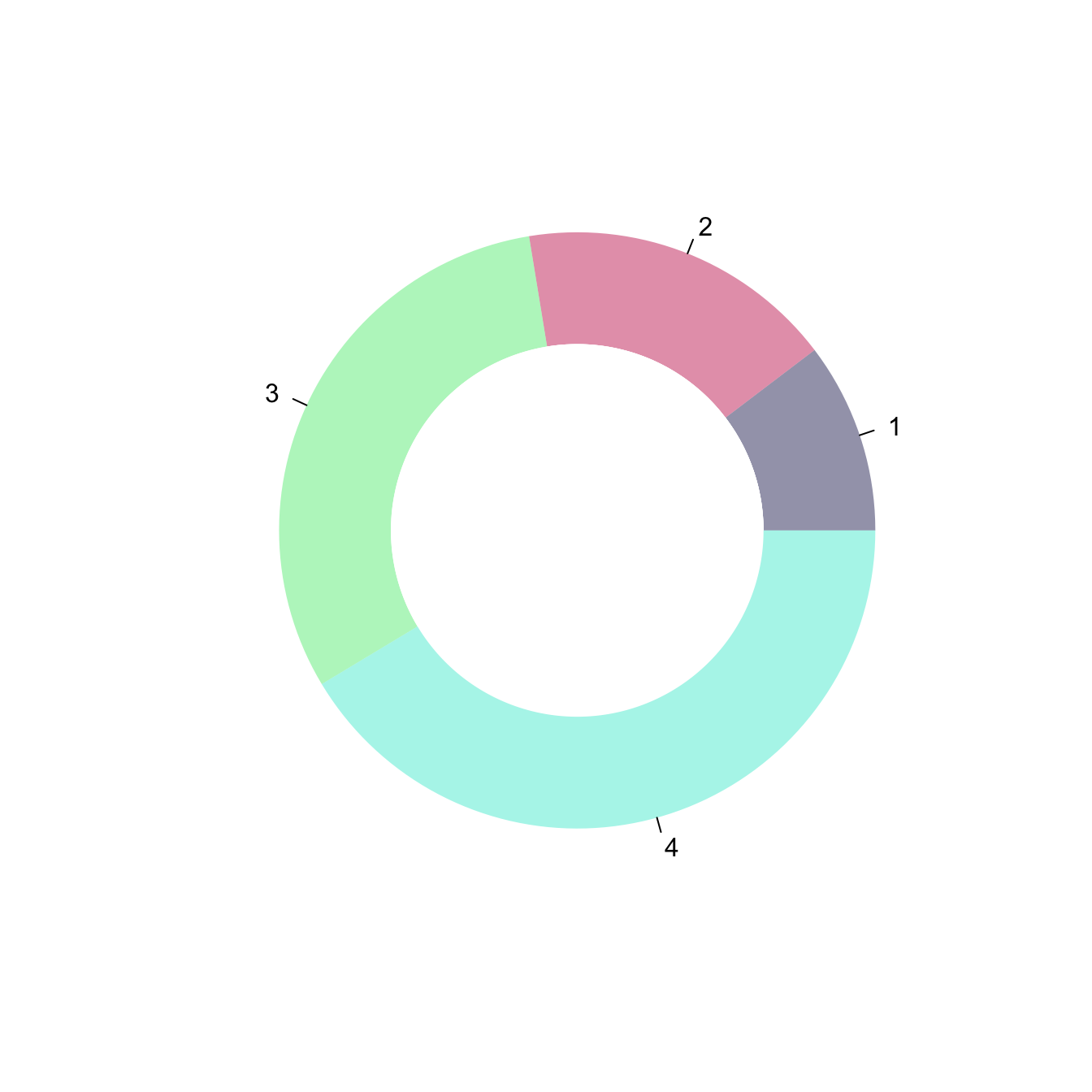# Donut chart with base R

This post provides code and explanation to build a donut chart in base R. It offers a `doughnut()` function that can be reused on any data.

To draw a donut plot, the easiest way is to use `ggplot2`, as suggested in graph #128.

If you want to stick to base R however, the function given below should allow you to get there.

Important: this functions comes from here.``````# The doughnut function permits to draw a donut plot
doughnut <-
function (x, labels = names(x), edges = 200, outer.radius = 0.8,
init.angle = if (clockwise) 90 else 0, density = NULL,
angle = 45, col = NULL, border = FALSE, lty = NULL,
main = NULL, ...)
{
if (!is.numeric(x) || any(is.na(x) | x < 0))
stop("'x' values must be positive.")
if (is.null(labels))
labels <- as.character(seq_along(x))
else labels <- as.graphicsAnnot(labels)
x <- c(0, cumsum(x)/sum(x))
dx <- diff(x)
nx <- length(dx)
plot.new()
pin <- par("pin")
xlim <- ylim <- c(-1, 1)
if (pin[1L] > pin[2L])
xlim <- (pin[1L]/pin[2L]) * xlim
else ylim <- (pin[2L]/pin[1L]) * ylim
plot.window(xlim, ylim, "", asp = 1)
if (is.null(col))
col <- if (is.null(density))
palette()
else par("fg")
col <- rep(col, length.out = nx)
border <- rep(border, length.out = nx)
lty <- rep(lty, length.out = nx)
angle <- rep(angle, length.out = nx)
density <- rep(density, length.out = nx)
twopi <- if (clockwise)
-2 * pi
else 2 * pi
t2p <- twopi * t + init.angle * pi/180
}
for (i in 1L:nx) {
n <- max(2, floor(edges * dx[i]))
P <- t2xy(seq.int(x[i], x[i + 1], length.out = n),
polygon(c(P\$x, 0), c(P\$y, 0), density = density[i],
angle = angle[i], border = border[i],
col = col[i], lty = lty[i])
Pout <- t2xy(mean(x[i + 0:1]), outer.radius)
lab <- as.character(labels[i])
if (!is.na(lab) && nzchar(lab)) {
lines(c(1, 1.05) * Pout\$x, c(1, 1.05) * Pout\$y)
text(1.1 * Pout\$x, 1.1 * Pout\$y, labels[i],
xpd = TRUE, adj = ifelse(Pout\$x < 0, 1, 0),
...)
}
Pin <- t2xy(seq.int(0, 1, length.out = n*nx),
polygon(Pin\$x, Pin\$y, density = density[i],
angle = angle[i], border = border[i],
col = "white", lty = lty[i])
}

title(main = main, ...)
invisible(NULL)
}

# Let's use the function, it works like PiePlot !
# inner.radius controls the width of the ring!
doughnut( c(3,5,9,12) , inner.radius=0.5, col=c(rgb(0.2,0.2,0.4,0.5), rgb(0.8,0.2,0.4,0.5), rgb(0.2,0.9,0.4,0.4) , rgb(0.0,0.9,0.8,0.4)) )``````

Related chart types

## Contact

This document is a work by Yan Holtz. Any feedback is highly encouraged. You can fill an issue on Github, drop me a message on Twitter, or send an email pasting yan.holtz.data with gmail.com.